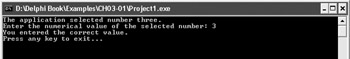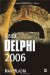The if-then Statement

The if-then statement is used to test an expression and see if the expression evaluates to True or False. Subsequent execution depends on the result of the if-then statement. If the expression evaluates to True, the statement or statements that follow are executed, and if the expression evaluates to False, the statement or statements that follow are skipped.

The syntax of the if-then statement is:

if expression then    statement;

To create a valid expression that can be used with the if-then statement, you usually have to use one or more relational operators. Table 3-1 contains the relational operators available in Delphi.

Table 3-1: Relational operators

Relational Operator

Meaning

=

Equality

<>

Inequality

<

Less than

>

Greater than

<=

Less than or equal to

>=

Greater than or equal to

The final result of all relational operators is a Boolean value, and thus the expression used in the if-then statement can be either an expression built with relational operators or a simple Boolean value.

Let's first see how to create an application that tests if the user has entered the correct value. The application will spell out a number and the user has to enter the correct numerical value. To keep things simple, the application always spells out the number three and successfully executes if the user enters 3 (see Figure 3-1).Figure 3-1: The result of the if-then statement

Listing 3-1: A simple if-then statementprogram Project1; {\$APPTYPE CONSOLE} uses   SysUtils; var   TheNumber: Integer; begin   WriteLn('The application selected number three.');   Write('Enter the numerical value of the selected number: ');   ReadLn(TheNumber);   if TheNumber = 3 then     WriteLn('You entered the correct value.');   WriteLn('Press any key to exit...');   ReadLn; end.The if-then statement is used to test the value the user entered into the TheNumber variable. If the user entered 3, the expression TheNumber = 3 evaluates to True and the application executes the line that displays the "You entered the correct value." message. If the user enters any other number, the application skips this line and executes the line that displays the "Press any key to exit…" message.

If you indent code properly, it's really easy to see which lines are executed depending on the result of the if-then statement. You should always try to follow the standard Borland formatting style since it is used in the Delphi documentation, in this book, and by the vast majority of Delphi programmers. Even with a superficial look at the source code in Listing 3-1, you can easily see the lines that belong to the main block since they are indented. The conditional WriteLn statement is also easy to spot since it is indented even more.

This example only executes one line of code if the expression evaluates to True. If you want to execute more than one line, you have to write them inside a block. All blocks begin with the reserved word begin, but only the main block ends with the reserved word end followed by a period. Other blocks have to end with the reserved word end followed by a semicolon:

if TheNumber = 3 then begin   WriteLn('You entered the correct value.');   WriteLn('Thank you for using this application.'); end;

When the if-then statement is followed by a block, all statements in the block are executed if the condition in the if-then statement evaluates to True.

If we want the application to display a message when the user enters an invalid value, we can use another if-then statement but with the opposite relational operator. In this case, we have to use the inequality (<>) operator to see if the user entered anything but the requested number 3.

Listing 3-2: Two if-then statementsprogram Project1; {\$APPTYPE CONSOLE} uses   SysUtils; var   TheNumber: Integer; begin   WriteLn('The application selected number three.');   Write('Enter the numerical value of the selected number: ');   ReadLn(TheNumber);   if TheNumber = 3 then     WriteLn('You entered the correct value.');   if TheNumber <> 3 then     WriteLn('Sorry. You entered the wrong value.');   WriteLn('Press any key to exit...');   ReadLn; end.In this example, we have manually inverted the relational operator to execute a piece of code if the user enters the wrong value. Although you can write two if-then statements to cover both the True and False results of the desired condition, this is usually done with another version of the if-then statement: the if-then-else statement.Inside Delphi 2006 (Wordware Delphi Developers Library)
ISBN: 1598220039
EAN: 2147483647
Year: 2004
Pages: 212